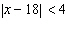Name:    Chapter 3 Pre Test

Multiple Choice
Identify the choice that best completes the statement or answers the question.

1.

A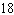-foot board is cut into three pieces. Two pieces have the same length and the third is twice as long as the others. How long are the pieces?
 a. Two pieces have lengthfeet and one piece has length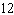feet. b. Two pieces have lengthfeet and one piece has lengthfeet. c. Two pieces have lengthfeet and one piece has length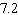feet. d. Two pieces have lengthfeet and one piece has length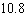feet. e. Two pieces have lengthfeet and one piece has length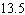feet.

2.

Solve the equationfor x. Round your answer to two decimal places.
 a.b.c.d.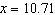e.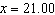3.

Convert the fractionto a percent.
 a. 1.22% b. 12.2% c. 122% d. 61% e. 3050%

4.

Complete the table showing the equivalent forms of a percent. Reduce fractions to simplest form.

 Percent Parts out of 100 Decimal Fraction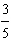a.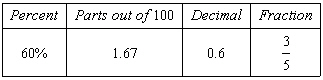b.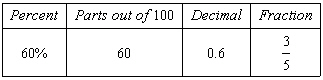c.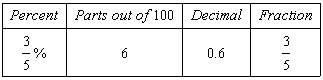d.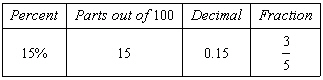e.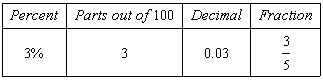5.

A hardware store is having a 35%-off sale. If an item has a list price of \$16.20, what is the item's sale price?
 a. 21.60% b. 21.87% c. 5.67% d. 10.53% e. 18.80%

6.

Find the unit price (in dollars per ounce) if a 1-pound, 9-ounce loaf of bread costs \$2. Round your answers to two decimal places.
 a. \$0.13 per ounce b. \$0.08 per ounce c. \$0.06 per ounce d. \$0.15 per ounce e. \$0.11 per ounce

7.

Solve the proportion.
 a.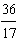b.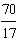c.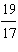d.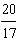e.8.

Solve the proportion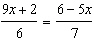to determine x.
 a.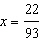b.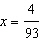c.d.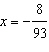e.9.

Suppose a car uses 60 gallons of gasoline for a trip of 600 miles. How many gallons would be used on a trip of 100 miles?
 a. 20 gallons b. 1000 gallons c. 21 gallons d. 10 gallons e. 1001 gallons

10.

Find the interest rate r given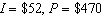and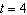years. Round your answer to the nearest integer.
 a. 1880% b. 208% c. 5% d. 3% e. 7%

11.

If an object traveled 230 miles at a rate of 25 miles per hour, how long (in hours) did it take to travel this distance?
 a. 9.7 hours b. 0.11 hour c. 5750 hours d. 9.2 hours e. 7.7 hours

12.

Find the surface area of the rectangular solid.
(Note: This is the combined area of the six surfaces.) Include the units in the answer.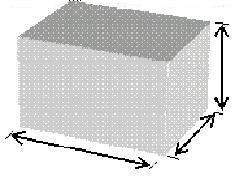13 in. 9 in.4 in.

 a.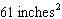b.c.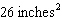d.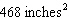e.13.

Suppose \$7000 is divided between two investments earning 9% and 5% simple interest. Over the course of a year the two accounts earned a total of \$554. How much was invested in each account?
 a. 1900 in the account earning 9%5100 in the account earning 5% b. 4600 in the account earning 9%2400 in the account earning 5% c. 5100 in the account earning 9%1900 in the account earning 5% d. 3500 in the account earning 9%3500 in the account earning 5% e. 2400 in the account earning 9%4600 in the account earning 5%

14.

Which of the following is a solution to the inequality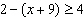?
 a.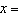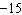b.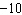c.d.e.15.

Which inequality has the graph that is shown?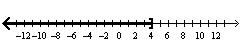a.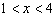b.c.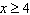d.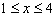e.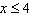16.

Graph the inequality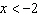or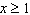.
 a.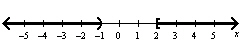b.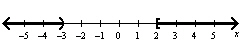c.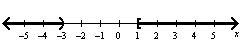d.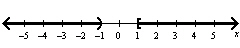e.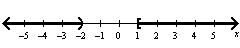17.

Determine whether the inequalities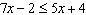and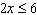are equivalent.
 a. equivalent b. not equivalent

18.

Determine if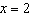is a solution to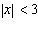.
 a.is a solution. b.is not a solution.

19.

Solve, if possible. Write the answer in set notation.
 a.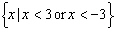b.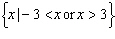c.d.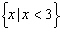e. no solution

20.

Write an absolute value inequality that represents the interval shown in the following figure.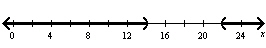a.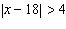b.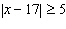c.d.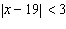e.